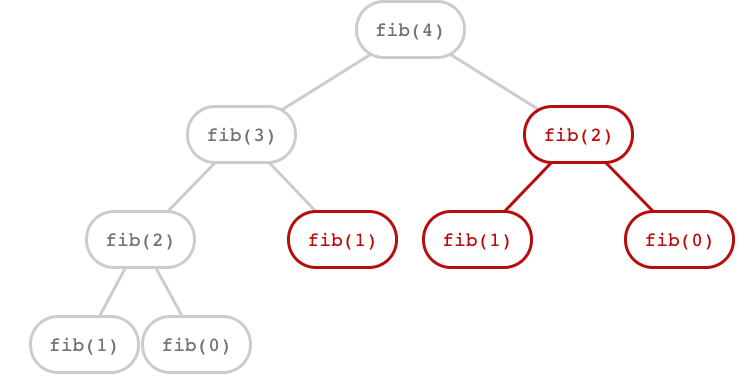# Tree Recursion

Tips for navigating the slides:
• Press O or Escape for overview mode.
• Visit this link for a nice printable version
• Press the copy icon on the upper right of code blocks to copy the code

### Class outline:

• Order of recursive calls
• Tree recursion
• Counting partitions

## Order of recursive calls


if n < 10:
print(n)
else:
print(n)
print(n)


What would this display?





if n < 10:
print(n)
else:
print(n)
print(n)


• Until the Return value appears, that call has not completed.
• Any statement can appear before or after the recursive call.
Global frame
 n 123 Return value None
 n 12 Return value None
 n 1 Return value None
Print output:

123
12
1
12
123



if n < 10:
print(n)
else:
print(n)
print(n)


print(n)
if n >= 10:
print(n)

• If two implementations are equally clear, then the shorter one is usually better
• When learning to write recursive functions, put the base cases first
• Both are recursive functions, even though only the first has typical structure


1
12
123
12
1



grow(n)
print(n)
shrink(n)

def f_then_g(f, g, n):
if n:
f(n)
g(n)


grow = lambda n: f_then_g(grow, print, n//10)
shrink = lambda n: f_then_g(print, shrink, n//10)


## Tree recursion

### Tree Recursion

Tree-shaped processes arise whenever a recursive function makes more than one recursive call.

Sierpinski curve

### Recursive Virahanka-Fibonacci

The nth number is defined as:

$$\small\begin{equation*} \text{virfib}(n) = \begin{cases} 0 & \text{if } n = 0 \\ 1 & \text{if } n = 1 \\ \text{virfib}(n - 1) + \text{virfib}(n - 2) & \text{otherwise} \\ \end{cases} \end{equation*}$$


def virfib(n):
"""Compute the nth Virahanka-Fibonacci number, for N >= 1.
>>> virfib(2)
1
>>> virfib(6)
8
"""
if n == 0:
return 0
elif n == 1:
return 1
else:
return virfib(n-2) + virfib(n-1)


### Redundant computations

The function is called on the same number multiple times. 🙀(We will speed up this computation dramatically in a few weeks by remembering results)

## Counting partitions

### Counting partitions problem

The number of partitions of a positive integer n, using parts up to size m, is the number of ways in which n can be expressed as the sum of positive integer parts up to m in increasing order.

count_partitions(6, 4)
 2 + 4 = 6 1 + 1 + 4 = 6 3 + 3 = 6 1 + 2 + 3 = 6 1 + 1 + 1 + 3 = 6 2 + 2 + 2 = 6 1 + 1 + 2 + 2 = 6 1 + 1 + 1 + 1 + 2 = 6 1 + 1 + 1 + 1 + 1 + 1 = 6

### Counting partitions approach

The number of partitions of a positive integer n, using parts up to size m, is the number of ways in which n can be expressed as the sum of positive integer parts up to m in increasing order.

count_partitions(6, 4)

Recursive decomposition: finding simpler instances of the problem.

Explore two possibilities:

• Use at least one 4
• Don't use any 4

Tree recursion often involves exploring different choices.

### Counting partitions approach

The number of partitions of a positive integer n, using parts up to size m, is the number of ways in which n can be expressed as the sum of positive integer parts up to m in increasing order.

count_partitions(6, 4)

Solve two simpler problems:

 count_partitions(2, 4) count_partitions(n-m, m) count_partitions(6, 3) count_partitions(n, m-1)

### Counting partitions code

The number of partitions of a positive integer n, using parts up to size m, is the number of ways in which n can be expressed as the sum of positive integer parts up to m in increasing order.

count_partitions(6, 4)

Solve two simpler problems:

with parts of size m:

count_partitions(2, 4)
count_partitions(n-m, m)

without parts of size m:

count_partitions(6, 3)
count_partitions(n, m-1)

def count_partitions(n, m):
"""
>>> count_partitions(6, 4)
9
"""
if n == 0:
return 1
elif n < 0:
return 0
elif m == 0:
return 0
else:
with_m = count_partitions(n-m, m)
without_m = count_partitions(n, m-1)
return with_m + without_m